Name: ___________________Date:___________________

 Email us to get an instant 20% discount on highly effective K-12 Math & English kwizNET Programs!

### Grade 3 - Mathematics1.3 Multiplication Arrays

 Method: Step 1: Count the pictures in each row. Step 2: Multiply the number of patterns in each row by the total number of rows. Example: Use the figure to find 3 times 5.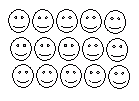This is made of 3 rows of 5 in each, we hence write as 5*3. Answer: 15 Directions: Answer the following questions also write at least ten examples of your own.
 Q 1: Use the figure to find 4 times 2.738 Q 2: Use the figure to find 1 times 5.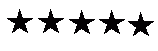567 Q 3: Use the figure to find 4 times 2.958 Q 4: Use the figure to find 3 times 2.756 Q 5: Use the figure to find 5 times 4.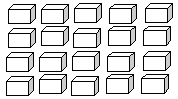192120 Q 6: Use the figure to find 3 times 2.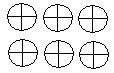746 Q 7: Use the figure to find 5 times 2.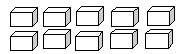101211 Q 8: Use the figure to find 5 times 2.8109 Question 9: This question is available to subscribers only! Question 10: This question is available to subscribers only!

#### Subscription to kwizNET Learning System costs less than \$1 per month & offers the following benefits:

• Unrestricted access to grade appropriate lessons, quizzes, & printable worksheets
• Instant scoring of online quizzes
• Progress tracking and award certificates to keep your student motivated
• Unlimited practice with auto-generated 'WIZ MATH' quizzes